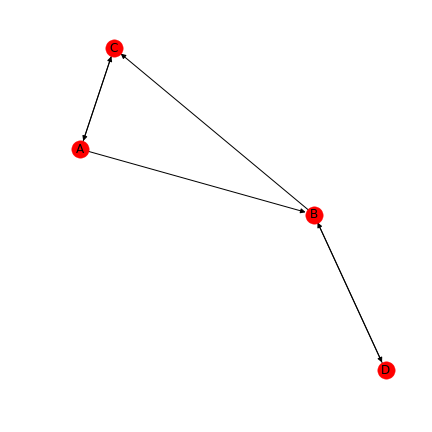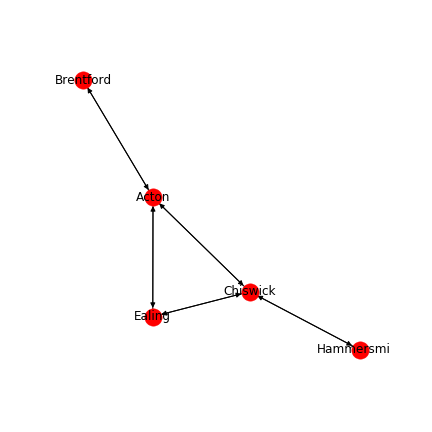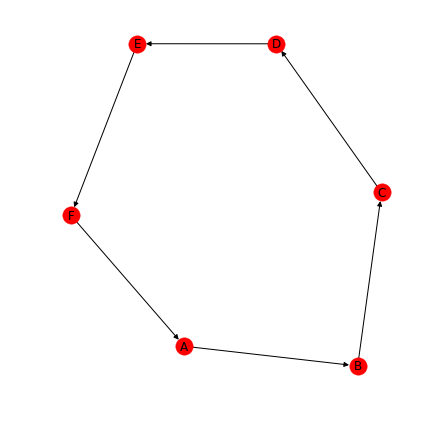# Basic Implementation of Graphs In Python

Posted on 11-02-19 in ComputingIn this article we will look at implementation of graphs in Python. First let's make sure we understand dictionaries!

# Dictionary Review¶

A Dictionary in Python is a data structure which relates unique keys to values. They are defined and indexed as such

In :
scores = {'mark' : 20, 'bob' : 15, 'mary' : 25}

scores['frank'] = 20  # new player

for player in scores:
print(f"{player.capitalize():<10} scores {scores[player]:3}")

Mark       scores  20
Bob        scores  15
Mary       scores  25
Frank      scores  20


Don't understand line 6? Review https://markbrowntuition.co.uk/computing/2017/11/10/fancy-printing-with-python/

Here we can iterate over dictionaries in the same way with lists. Importantly we need to remember that whilst in later versions of Python that dictionaries are ordered, this is not a guaranteed behaviour!

## Questions¶

In [ ]:
parcel = {'length' : 0, 'width' : 0, 'height' : 0, 'weight' : 0}

# 1 Iterate Over the parcel object and ask the user for values

# 2 Add new entries to the parcel for volume and density


## What is a graph?¶A graph is points (called nodes) connected by lines (called edges). These edges can be one-way or two-ways. We can also assign weights to these edges. These can correspond to whatever we need - the length of a road or the difficulty in getting from one PC to another.

In this article we will cover how to use Python to define such graphs and how to traverse them in various ways. We will use two possible representations of the graph

1. Dictionaries
2. Adjacency Matrix

Let's look at the first.

In :
simple_path = {'A' : 'B',
'B' : 'C',
'C' : 'D',
'D' : 'E',
'E' : 'F',
'F' : 'A'
}

for node in simple_path:
print(f"{node} is connected to {simple_path[node]}")

A is connected to B
B is connected to C
C is connected to D
D is connected to E
E is connected to F
F is connected to A


Here we have used the keys in the dictionary as the list of nodes available. The value in this case holds a single possible destination. I've drawn this graph above for a visual comparison.

This needs to be altered slightly - after all a single node can be connected to multiple places!

In :
simple_path = {'A' : ['B',],
'B' : ['C',],
'C' : ['D',],
'D' : ['E',],
'E' : ['F',],
'F' : ['A',]
}

for node in simple_path:
print(f"{node} is connected to {simple_path[node]}")

A is connected to ['B']
B is connected to ['C']
C is connected to ['D']
D is connected to ['E']
E is connected to ['F']
F is connected to ['A']


## Questions¶

1. Give at least three examples of a graphs.
2. The above graph is uni-directional. Alter it so we can go either direction around the loop.
3. Create a dictionary for the following images# Basics of Graphs¶

## 1: Simple Graph Traversal¶

A graph has nodes (locations) and edges connecting them. These can represent many things such as roads between towns or mazes.

We will begin with a simple representation using a dictionary and attempt to traverse this.

In :
simple_path = {'A' : ['B',],
'B' : ['C',],
'C' : ['D',],
'D' : ['E',],
'E' : ['F',],
'F' : ['A',]
}

to_visit = ['A', ]
visited = []

while to_visit:
current_location = to_visit.pop(0)
print(f"We are currently at {current_location}")
visited.append(current_location)
for node in simple_path[current_location]:
if node not in visited:
to_visit.append(node)

We are currently at A
We are currently at B
We are currently at C
We are currently at D
We are currently at E
We are currently at F


## Questions¶

1. What is the purpose of the visited list?
2. Add an end node. If we arrive at this node, end early.
3. Visit every node n times, not once.
4. From a list of nodes, create a dictionary that makes every node connected to every other node.
5. Traverse the incomplete graph below. How can we ensure the computer does not crash? Aside When would you want to visit a location several times?
In :
simple_path = {'A' : ['B',],
'B' : ['C',],
'C' : ['D',],
'D' : ['E',],
'E' : ['F', 'G'],
}

to_visit = ['A', ]
visited = []

while to_visit:
current_location = to_visit.pop(0)
print(f"We are currently at {current_location}")
visited.append(current_location)
for node in simple_path[current_location]:
if node not in visited:
to_visit.append(node)

We are currently at A
We are currently at B
We are currently at C
We are currently at D
We are currently at E
We are currently at F

---------------------------------------------------------------------------
KeyError                                  Traceback (most recent call last)
<ipython-input-37-c707402c755b> in <module>()
13     print(f"We are currently at {current_location}")
14     visited.append(current_location)
---> 15     for node in simple_path[current_location]:
16         if node not in visited:
17             to_visit.append(node)

KeyError: 'F'

## Aside Drawing Graphs in Python¶

In :
import networkx as nx
from matplotlib.pyplot import subplots, show
from matplotlib import style
simple_path = {'A' : 'B',
'B' : 'C',
'C' : 'D',
'D' : 'E',
'E' : 'F',
'F' : 'A'
}

fig, ax = subplots(figsize=(6,6))
g = nx.DiGraph(simple_path)
pos = nx.spring_layout(g)
nx.draw(g, pos=pos, ax=ax, with_labels=True, font_size=12)
fig.tight_layout()

fig.savefig('circular_graph.png')
show()